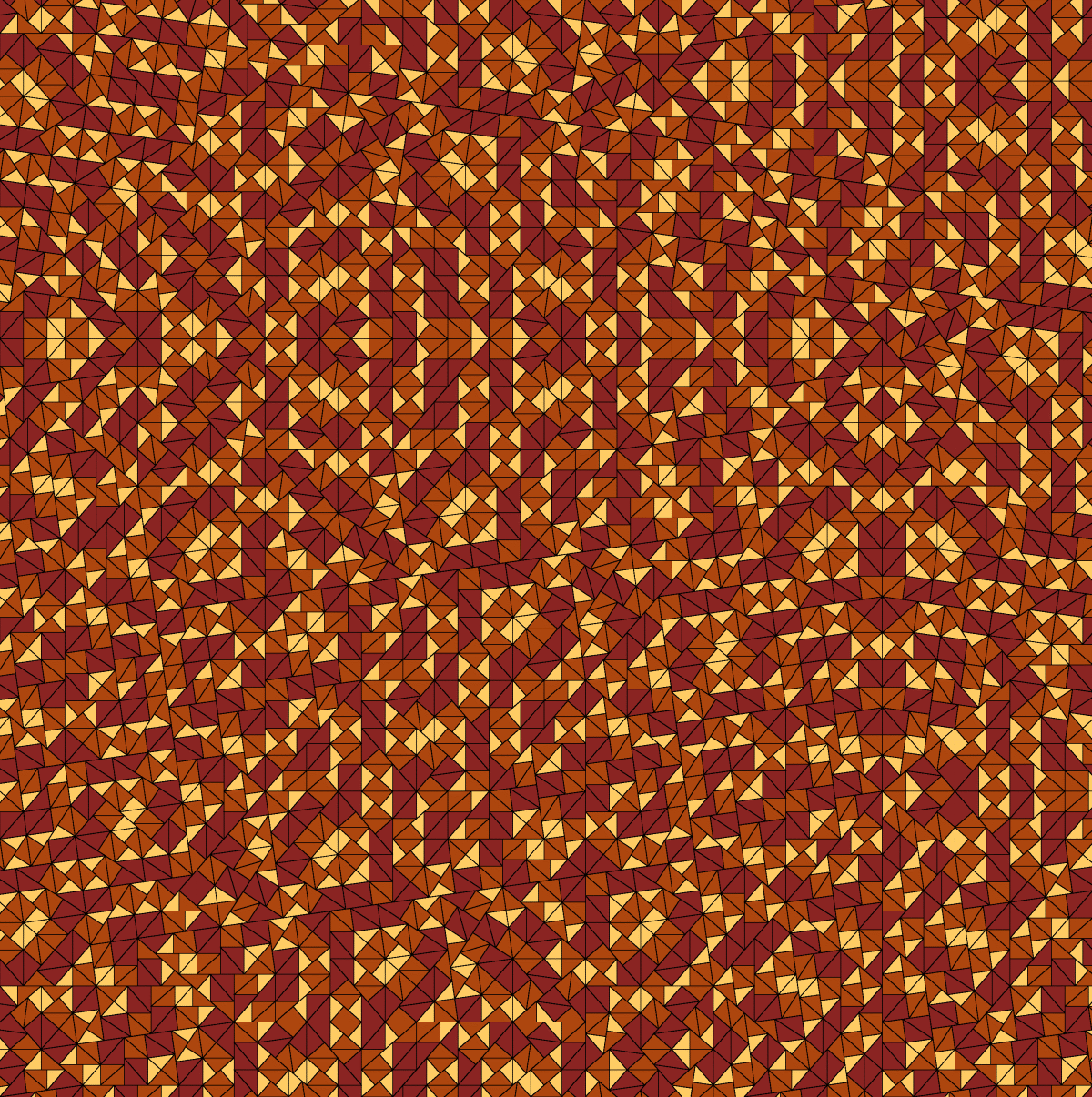## Pythia-3-1

### Info

A simple example of an infinite series of substitutions with tilings of statistical circular symmetry. It is shown in [Frettloeh:STWCS not found], that all tilings in this series posses statistical circular symmetry. The substitution factors are $s2m$, where s is the largest root of $xm-xk-1$. Each pair of integers $(m,k)$, where $m>k, m>2, k>0$, encodes a such a Pythia substitution. The case $m=4, k=2$ yields the golden pinwheel substitution.

### Substitution Rule### Patch